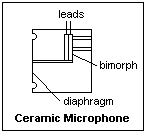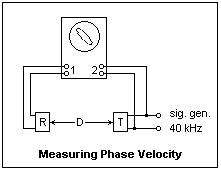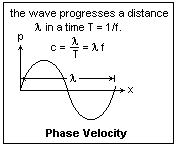## The Speed of Sound

Acoustics is a subject of great practical importance, and electronics plays a large role in the generation, detection and measurement of sound. On this page, the measurement of the speed of sound, and some topics that it brings up, will be described. This is not a general treatment of acoustics, of course, but only of a few topics that are interesting and are good general knowledge for the experimenter. Other pages on this website give further information on the physical and psychological aspects of sound.

### The Newton-Laplace Formula

The difference in the speeds of propagation of light and sound are evident from the casual observation of lightning. The sound follows the flash after a delay proportional to the distance, as if light propagated instantaneously (which is does, for all practical purposes) and sound at a speed of 1100 ft/s or 340 m/s under typical conditions. When we observe lightning, the paths of the sound rays are affected by the vertical temperature gradient (lapse rate), so usually the thunder from any considerable distance passes overhead.

This effect was used for one of the early determinations of the speed of sound. In 1709, Derham observed cannon firing on Blackheath from 12.5 miles away with a telescope, north over the Thames from Upminster Church tower. He timed the delay at from 55-63 s, which gave a speed of 1142 ft/s or 348 m/s, a pretty good result. Isaac Newton had determined theoretically in 1686 that the speed of sound should be the reciprocal of the square root of the product of the compressibility of air, κ, and the density, ρ, or c = 1/√κρ. We use c for the speed of sound. The compressibility is (1/V)(∂V/∂p) = 1/p for an ideal gas, where p is the pressure. This yields c = √(p/ρ). Unfortunately, this theory gave a value some 15% too low when compared with Derham's and other results. The discrepancy was too large to be explained by experimental error or inaccurate constants.

The mystery was not solved until 1816, when Laplace pointed out that the sound vibrations were too rapid to allow thermal equilibrium, and the variations in density were adiabatic, not isothermal, as Newton had assumed. The slight heating when a gas was compressed made it less compressible, which would raise the predicted speed of sound to the observed value. In fact, κS = κT/γ, where γ is the ratio of the specific heat at constant pressure to the specific heat of constant volume. For diatomic gases like air, at normal temperatures, γ = 1.40, approximately (for air, γ = 1.402). Laplace's formula, c = √(γp/ρ), agrees excellently with experiment.

Using the ideal gas law p = ρRT/M, where T is the absolute temperature in K, R is the universal gas constant 8314.32 J/K-kmol and M is the molecular weight in kg, we find c = √(γRT/M). Since the pressure and the density are proportional, the speed of sound is independent of pressure but proportional to the square root of the absolute temperature. This is the result for an ideal gas, but it holds very well for all gases at low pressure. The molecular weight of dry air is 28.966. Moist air has a lower density than dry air, so the speed of sound is a little faster in it, but the difference is quite small. At 0°C, c = 331.4 m/s, and at 20°C, c = 343.4 m/s. A value of 340 m/s is a good average value for normal conditions.

The speed of sound is remarkably dependent on the molecular weight of the gas. When helium (M = 4) is breathed, the speed of sound is so high that all the vocal resonances on which the pitch of the voice depends are raised in frequency, with the usual droll effect. Carbon dioxide (M = 44) would produce the opposite effect, but is not healthy to breathe in the necessary concentration. Nitrous oxide (M = 40) would produce a similar effect, but with few ill effects. The normal medium for sound is air, however, so these variations are uncommon.

### Ultrasonic TransducersIt turns out to be relatively easy to measure the speed of sound approximately with the facilities of modern electronics. Ultrasonic ceramic sensors are convenient for the purpose. A reasonable pair is manufactured by Panasonic and is available from Digi-Key as receiver P9894 and transmitter P9895. I used a cheaper pair from another distributor that seem to be similar. The transmitter and receiver are both resonant at about 40 kHz, and have little output or sensitivity away from this frequency. They use a ceramic element, which could be barium titanate (BaTiO3), that is strongly piezoelectric. They are similar to the ceramic microphones that contained a diaphragm-actuated ceramic "bimorph" that produced a signal when it was distorted. It would be interesting to know the exact construction of these sensors, but this information is not available to me. An idea of the construction of an ordinary ceramic microphone is shown at the right.

The transmitter has a metal case and a black fiber back, marked with a T, with two pins for the connections. The maximum voltage that can be applied is around 20 V, but I do not have specific information for the one I used, so I kept the voltage as low as possible (5 - 10 V peak-to-peak). The sound pressure level (SPL) produced by the transmitter is given as 105 dB. To use this figure, one must know what SPL is, and how the value was measured, but this information does not accompany the catalog entry.

The sound pressure level in dB is defined as SPL = 20 log10 (p/po), where p is the overpressure in the wave, and po is a reference pressure. Where human hearing is involved, po = 200 μb (a μb, microbar, is the same as 1 dyne/cm2, or 1 Pa). An SPL of 0 dB is just audible under the best conditions with this reference. For other purposes, po = 1 mb is used. A millibar (mb) is the usual unit. It is not clear which one is used here, but I suspect it is the latter.

With a reference pressure of 200 μb, ordinary conversation is SPL 70 dB, and anything over 140 dB is damaging. Of course, these figures are for audible frequencies (20 Hz - 10 kHz) only.

The receiver has an all-metal case. Like the transmitter, it is 16 mm in diameter and 12 mm long. The connections are made to two pins on the back, one soldered to the case. Its sensitivity is listed as -70 dBV/mb. Since the dBV is defined as dBV= 20 log10 V (reference value 1 V), this is the same as 0.315 mV/mb. This seems to be a middling value, between the -45 dB for a carbon microphone and the -85 dB for a moving-coil microphone. Again, the results will depend on the conditions of measurement. One might presume that this is the sensitivity when isolated in a free field that is not sensibly disturbed by the presence of the microphone. On the other hand, the specifications could be for the sensors in an infinite baffle.

In any case, the resonant nature of these sensors means that all of these figures are strongly dependent on frequency.

### Measuring the Speed of Sound

The sensors should be mounted securely. I put them in 5/8" holes bored in short pieces of 1 x 2. The transmitter was clamped to a length of 1 x 2 with a C-clamp, while the receiver was free to move back and forth, secured in any position with an elastic band. The distance from transmitter to receiver could be varied up to about 300 mm. This is certainly not ideal, but was fast and convenient. You will have no success without some such method of support.The transmitter was driven directly by the 600Ω output of a function generator, while the output of the receiver was examined by an oscilloscope probe, without any amplification. The setup is shown at the right. It is remarkable that this worked well enough, though some amplification would have been convenient. Connect the receiver to channel 2, and the output of the signal generator to channel 1. Observe the output of the sensor as the frequency is varied, and set the frequency for maximum response, which will be a compromise resonant frequency for the two sensors. This frequency will be quite close to 40 kHz. Use only sufficient transmitter voltage to produce a usable received signal.

Now arrange the oscilloscope for XY display. Adjust the gains so that the ellipse has axes at as close to 45° as possible, and varies from lines to a circle as the receiver is moved. What we are looking at is the phase difference between the signal applied by the function generator and the signal received by the sensor. When the sensor is moved so that a complete cycle of Lissajous figures has occurred, it has moved one wavelength. I quickly found a wavelength to be about 8 mm. This gives c = 40 kHz x 8 mm = 320 m/s, which is satisfactory.

This experiment can be carried out equally well with a pair of loudspeakers with matching (audio output, 5000Ω - 8Ω) transformers. In this form, it is a classic.This measurement, based on the variation of phase with distance, is the phase velocity, obeying the familiar formula c = fλ, which is illustrated at the left. A wave front propagates normal to itself at the phase velocity. This explains very clearly why a sound wave is bent upwards if it is cooler aloft, as is generally the case, so that sounds are generally not heard at large distances. If the phase velocity varies with the frequency, the medium is called dispersive. For ordinary sound waves in air, the speed of sound does not depend on the frequency, so the propagation is nondispersive. This means that the different frequency components of a complex signal do not get out of step, and the shape of the wave is preserved. For very strong sound waves, this is no longer true. The higher frequencies propagate faster, and catch up with the lower ones, producing a shock wave of typical form.

We could also measure the sound speed with a sharp pulse or impulse, rather than a wave of definite frequency (but not with these resonant sensors!). In this case, we would be measuring the group velocity, which can differ from the phase velocity. If the medium is nondispersive, then the phase and group velocities are the same. This is generally the case with sound, so we can measure the sound velocity either way. Our sensors give phase velocity, Derham's cannon group velocity, but they will be the same.

We varied the distance in our measurement. It is also possible to vary the frequency. If we have a path of fixed length D, then if N waves just fit for a frequency f1, N + 1 will jut fit for a slightly higher frequency f2. Then, a little algebra shows that c = D(f2 - f1). If we try this with our sensors, taking D as the distance between them, and using the oscilloscope to detect changes, we do not get very good results. The reason is that there is an unknown and variable difference in phase between the applied voltage and the acoustic pressure of the transmitter, due to the effects of resonance.

If we wish to make more accurate measurements, the easiest way would be to move the sensor a number of wavelengths, and then divide the total distance by the number of wavelengths. With my crude apparatus, it is difficult to count the wavelengths, but it would be quite possible if there were a better way to move the sensor steadily. This would improve our results by about a factor of 10 or 20. Actually, knowing the approximate wavelength, it would not be necessary to count each wavelength. The total distance would give the approximate number of wavelengths, and the exact position of a node would give the distance. Procedures like this, but in reverse, are often used in laser determinations of distance.

It becomes evident that the exact determination of the speed of sound, to, say one part in ten thousand, is not a simple task. It depends on the elimination of various sources of error and bias. One apparatus for accurate measurement is the acoustic interferometer, where the distance between fixed reflectors is varied. There are very sharp resonances in this case. Such methods are required to study the dispersion of acoustic waves.